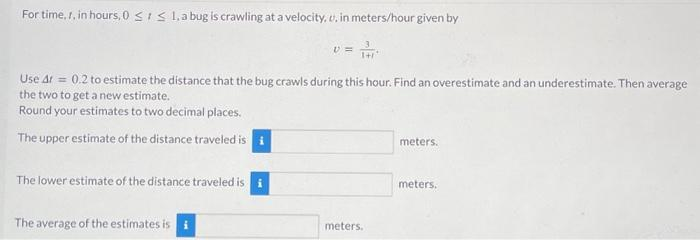Home / Expert Answers / Calculus / for-time-t-in-hours-0-t-1-a-bug-is-crawling-at-a-velocity-u-in-meters-hour-given-by-3-pa970

# (Solved): For time, t, in hours, 0 t 1, a bug is crawling at a velocity, u, in meters/hour given by 3 ...

For time, t, in hours, 0 ≤ t ≤ 1, a bug is crawling at a velocity, u, in meters/hour given by 3 1+1 Use At = 0.2 to estimate the distance that the bug crawls during this hour. Find an overestimate and an underestimate. Then average the two to get a new estimate. Round your estimates to two decimal places. The upper estimate of the distance traveled is The lower estimate of the distance traveled is i V= The average of the estimates is meters. meters. meters.For time, , in hours, , a bug is crawling at a velocity, , in meters/hour given by Use to estimate the distance that the bug crawls during this hour. Find an overestimate and an underestimate. Then average the two to get a new estimate. Round your estimates to two decimal places. The upper estimate of the distance traveled is meters. The lower estimate of the distance traveled is meters. The average of the estimates is meters.

We have an Answer from Expert